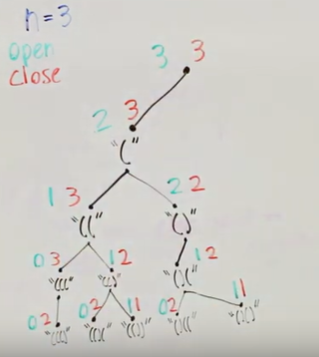# Back to Backtracking

Backtracking is a concept to build a solution using recursion,going through all states and rejecting the state which did not satisfy the constraints.for understanding we will solve problem name:

Generate all N matched parentheses.

INPUT is :Integer N

OUTPUT is :string of parentheses “(” or “)”…the string should be balanced.
By BALANCED I mean(number of “(” open== number of “)” closing brackets)

So for input N:::

N=1 output is [" () "]
{note 1: opening should be before closing}

N=2 output is [ " (()) “,” ()() "]
{note 2: we have to print all possible solution permutation}

N=3 output is [" ((())) “,” (())() “,” ()()() ", " (()()) “,”()(()()) "]

The three key to recursion

1. Our Choice
{either use ( or ) }
2.Our constraints
{we can not use “)” before “(”}
3:Our Goal
{total 2*N character should be used N ->" ( "+ N -> “)” }

now we move step by step to solve the problem for N=3When the number of open “(“ bracket is zero we start decreasing count of closing brackets “)” or when open < close

We gonna approach by taking input N and using a recursive function Back_track() with Parameter:

Back_track( number of “(” opening bracket
, number of* “)” closing bracket ,
Empty string which will be filled in each recursion step with either ( or )
vector of string to store the filled strings )**

:::::::::::::::::::CODE:::::::::::::::::::::::::::::::::

class Solution {
public:
void Back_track(int open,int close,string& bracket,vector&v){
if(open==0 && close==0)
{
v.push_back(bracket);
return;
}
if(open>0){
Back_track(open-1, close, bracket + ’(‘ , v );
}
if(open<close){
Back_track(open , close-1 , bracket + ’)’ , v );
}

`````` }

vector<string> generateParenthesis(int n) {

string bracket=””;
int open=n,close=n;
vector<string>v;
Back_track(open,close,bracket,v);
return v;

}
};``````
5 Likes

Thank You:+1:. This is really helpful for beginners1 Like

I suck at backtracking so much … Thanks !

2 Likes

You are so humble2 Likes